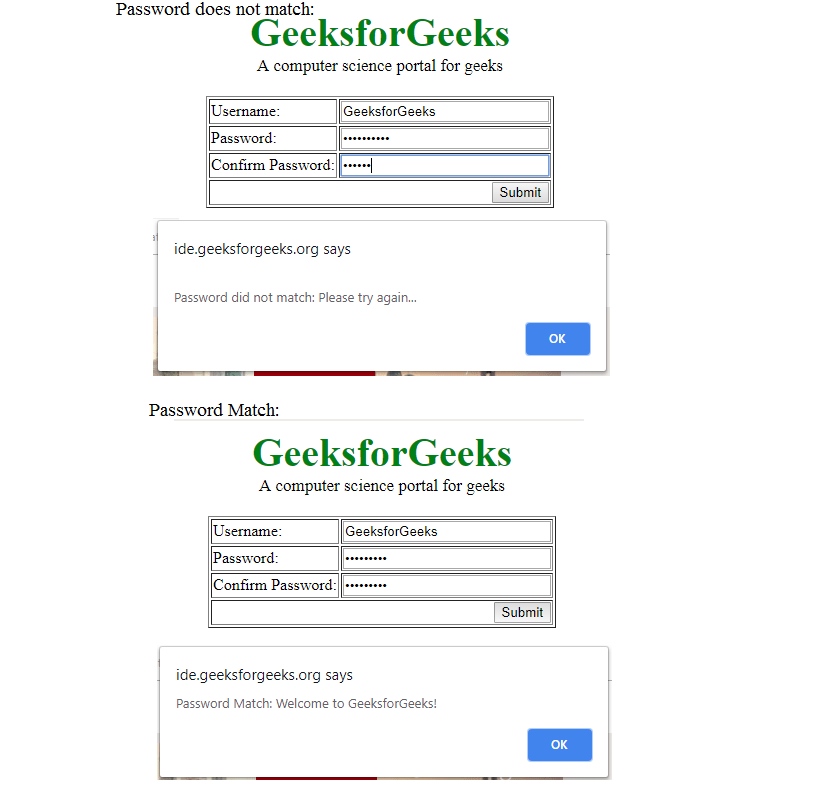It is used in online application form or social sites to signup account to verify the entered password by user is correct or not. It is the simple method to verify the password matches. First password is stored into a password1 variable and confirm password is stored in password2 variable. Then check if both variable value is equal then password match otherwise password does not match.

Below is the implementation of above approach:

 ` ` `<``html``> ` `    ``<``head``> ` `        ``<``script``> ` `         `  `            ``// Function to check Whether both passwords ` `            ``// is same or not. ` `            ``function checkPassword(form) { ` `                ``password1 = form.password1.value; ` `                ``password2 = form.password2.value; ` ` `  `                ``// If password not entered ` `                ``if (password1 == '') ` `                    ``alert ("Please enter Password"); ` `                     `  `                ``// If confirm password not entered ` `                ``else if (password2 == '') ` `                    ``alert ("Please enter confirm password"); ` `                     `  `                ``// If Not same return False.     ` `                ``else if (password1 != password2) { ` `                    ``alert ("\nPassword did not match: Please try again...") ` `                    ``return false; ` `                ``} ` ` `  `                ``// If same return True. ` `                ``else{ ` `                    ``alert("Password Match: Welcome to GeeksforGeeks!") ` `                    ``return true; ` `                ``} ` `            ``} ` `        `` ` `        ``<``style``> ` `            ``.gfg { ` `                ``font-size:40px; ` `                ``color:green; ` `                ``font-weight:bold; ` `                ``text-align:center; ` `            ``} ` `            ``.geeks { ` `                ``font-size:17px; ` `                ``text-align:center; ` `                ``margin-bottom:20px; ` `            ``} ` `        `` ` `    `` ` `    ``<``body``> ` `        ``<``div` `class` `= ``"gfg"``>GeeksforGeeks ` `        ``<``div` `class` `= ``"geeks"``>A computer science portal for geeks ` `        ``<``form` `onSubmit` `= ``"return checkPassword(this)"``> ` `        ``<``table` `border` `= ``1` `align` `= ``"center"``> ` `            ``<``tr``> ` `                ``` `                ``<``td``>Username: ` `                ``<``td``><``input` `type` `= ``text` `name` `= name ``size` `= ``25`` ` `            `` ` `            ``<``tr``> ` `                ``` `                ``<``td``>Password: ` `                ``<``td``><``input` `type` `= ``password` `name` `= ``password1` `size` `= ``25`` ` `            `` ` `            ``<``tr``> ` `                ``` `                ``<``td``>Confirm Password: ` `                ``<``td``><``input` `type` `= ``password` `name` `= ``password2` `size` `= ``25``> ` `            `` ` `            ``<``tr``> ` `                ``<``td` `colspan` `= ``2` `align` `= ``right``> ` `                ``<``input` `type` `= ``submit` `value` `= ``"Submit"``> ` `            `` ` `        `` ` `        `` ` `    `` ` `                     `

Output:My Personal Notes arrow_drop_up

## Recommended Posts:

Article Tags :

Be the First to upvote.

Please write to us at contribute@geeksforgeeks.org to report any issue with the above content.